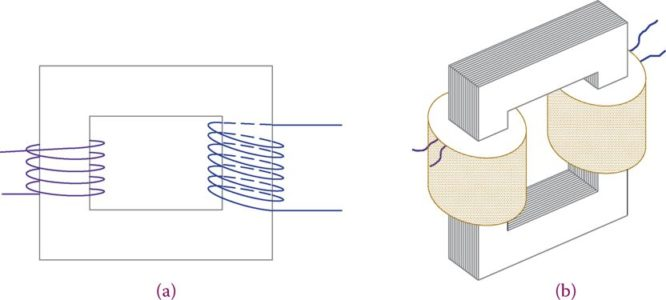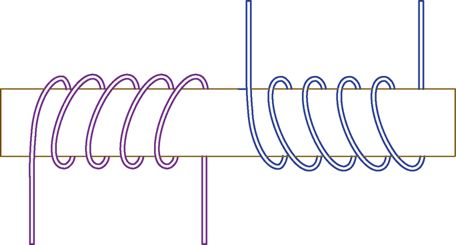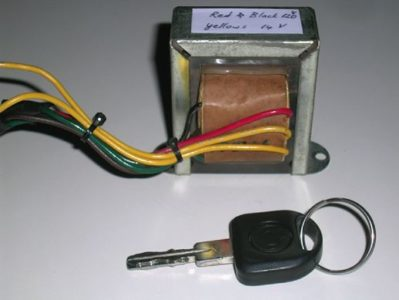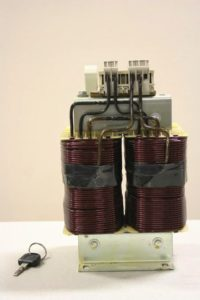Home / Transformer / Single-Phase Transformer | Construction | Theory

# Single-Phase Transformer | Construction | Theory

Want create site? Find Free WordPress Themes and plugins.

In the same way that single-phase AC and three-phase AC are different, although both are alternating current electricity, single-phase and three-phase transformers cannot be interchanged. Nevertheless, most of what can be said for single-phase transformers is later on extended to three-phase transformers because they work based on the same principle.

Transformers work based on the mutual induction between two windings. When electricity is applied to a coil a magnetic field is developed. Because in AC the voltage (and thus the current) continuously alters, the resulting magnetic field is variable. However, if a coil is placed in a varying magnetic field, it is similar to a wire that moves in a magnetic field; then a voltage is induced in it.

## Construction of Single-Phase Transformer

In the simplest form, a single-phase transformer is made up of two windings which share a common core. The core must be closed and its material is a ferromagnetic metal; it can be of various shapes, but the shape does not play a significant role.

Some shapes are preferable to the others, either because of manufacturing or because they can result in a better performance. The most common shapes for a core are rectangular and circular.

From the manufacturing viewpoint, a rectangular shape is easier to work with. Figure 1shows a transformer with a rectangular core. In this configuration, called a core-type transformer, the two windings are on the opposite sides (legs) of the core. This is not necessary, and both can be on the same leg.

In fact, most of the small transformers have a core as shown in Figure 2, and both windings are placed—usually, side by side—on the middle leg. This is a called a shell-type transformer.

Core-type transformer: Category for the construction of a transformer in which the core has the shape of a rectangular frame, and the windings are normally on the opposite sides of the rectangle.

Shell-type transformer: Category for the construction of a transformer in which the core has the shape of figure 8 (i.e., with a bridge in the middle of a rectangular frame).Figure 1 Basic structure of a single-phase transformer (core type). (a) Schematics. (b) Pictorial representation.

The two windings are called “primary” and “secondary,” depending on their role. The primary winding is connected to the voltage that we want to change. The secondary winding provides the required voltage.

In other words, the primary winding is connected to the input voltage and the secondary winding is the output and is connected to the output circuitry. To show a transformer in a circuit, the transformer symbol is used, as depicted in Figure 3.Figure 2 Most of transformers have a shell-type structure. (a) Schematics of a shell-type transformer. (b) Pictorial view.

If the primary winding has more turns than the secondary winding, the transformer decreases the voltage and it is a step-down transformer. If the primary winding has fewer turns than the secondary winding, the output voltage is higher than the input voltage and the transformer is called a step-up transformer.Figure 3 Symbol for a transformer.

The numbers of wire turn in the primary and secondary windings are represented by N1 and N2, respectively.

Step-down transformer: Transformer with secondary winding having fewer turns than the primary winding, thus decreasing voltage.

Step-up transformer: Transformer with secondary winding having more turns than the primary winding, thus increasing voltage.

For a step-down transformer, N1 > N2 and V1 > V2.

For a step-up transformer, N1 < N2 and V1 < V2.

Note that a wire can be wound around a core in two directions, as shown in Figure 4. As a result, depending on the directions of both windings in a transformer, at a given instant and compared to the primary voltage, the current in the secondary winding can be in one or the opposite direction.Figure 4 Two possible ways of winding a wire around a core.

In other words, the secondary voltage can be in phase with the primary voltage, or it can be 180° out of phase with it. It is, therefore, important to pay attention to the direction of turns in the winding of a transformer.

In Figure 2, for instance, both windings have the same direction. To show the polarity in the drawings of transformer circuits, a dot is put at one side of each winding indication the phase relationship, illustrating also the relative directions of the primary and secondary currents. This is indicated in Figure 5.Figure 5 Number of turns N, voltage V and current I in the primary and secondary circuits of a transformer.

Thus, for example, considering a sinusoidal waveform, when the side with a dot in the primary winding is at its maximum value the side with a dot in the secondary winding is at its maximum value.

In practice, the terminals are marked by letters such as H and X on the primary and secondary referring to the dot side to indicate this polarity. Figure 5 also indicates the number of turns N, the voltage V, and the current I and its direction for a given instant, identified by subscripts 1 and 2 for the primary and the secondary circuits, respectively.

## Multi-Output Transformers

It is possible to have multiple secondary windings on the same transformer. This provides various voltages from one source voltage, thus reducing the cost and space. This is very common in electronic devices such as a television that need various voltages for their operation. This is done in very small transformers.

In larger transformers and three-phase transformers, it is not normally necessary to have multiple voltages. Figure 6 shows the schematics of a multi-output transformer.Figure 6 Multi-output transformer.

Figure 7 shows a very small, 20 W, transformer. It converts 120 V into 6 and 12 V. In this sense, it is a two-output transformer. A 4 kVA industrial transformer is shown in Figure 8.Figure 7 A 20 W single-phase transformer.Figure 8 A 4 kVA single-phase transformer.

Did you find apk for android? You can find new Free Android Games and apps.

### About Ahmad FaizanMr. Ahmed Faizan Sheikh, M.Sc. (USA), Research Fellow (USA), a member of IEEE & CIGRE, is a Fulbright Alumnus and earned his Master’s Degree in Electrical and Power Engineering from Kansas State University, USA.